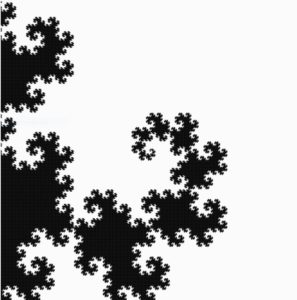# Concept

For this assignment, I have created multiple “glitchy” dragon curves, rotated with respect to the canvas center. The dragon curve is a widely known space-filling curve, meaning multiple of these curves can cover the entire 2D plane without overlapping. Not to mention, the curve is a fractal!  I first encountered this curve in my freshman year in high school, and the experience was mesmerizing. For this reason, I decided to draw dragon curves as soon as I read the assignment description.  Although the curve alone is indeed awe-inspiring, I added a glitchy appearance to the curves to make them look more interesting. The picture below is a single dragon curve without a glitchy appearance.

#Code

I spent most of the time finding and coding an algorithm to create dragon curves. Fortunately, the algorithm was very intuitive because dragon curves are formed by “folding” a line segment 90° in left or right directions. I first declared an array (dirArr) that stores these directional data, which describes how line segments will be folded.

`let dirArr = []; //Direciton Array storing Left or Right turn for each line segments`

A function was created to fill this array using a recursive algorithm I have coded (1 indicates a right turn and -1 indicates a left turn). The default size of dirArr is 1, and it contains 1, meaning a right turn. As iteration number increases, dirArr will store more directional data, making curve more complex. The algorithm fills dirArr in this way:

 -> [1, 1,-1] -> [1,1,-1,1,1,-1,-1] -> [1,1,-1,1,1,-1,-1,1,1,1,-1,-1,1,-1,-1]

Each array represents directional data stored in dirArr of different iterations.

1. For each iteration, the algorithm adds 1 at the end.
2. Then, directional data that were in the previous iteration’s dirArr (red) are copied into a temporary array.
3. Copied data in the temporary array are reversed (1 to -1 and -1 to 1). This data will then be added to dirArr in reverse order (blue).
4. Steps 1,2, and 3 will be done until given iteration of dirArr is achieved (recursion).
```function fillDirArr(iteration, myArr) {
//Store Left or Right turns into dirArr. 1 = Right turn, -1 = Left
count++;
let tempArr = myArr.slice(); //tempary array to store dirArr
myArr.push(1);
let tempArrLength = tempArr.length;
for (let i = 0; i < tempArrLength; i++) {
let currDir = tempArr.pop(); //Read tempArr from the end
myArr.push(-1 * currDir); //Reverse direction and push to currDir
}
if (iteration == count) {
count = 0;
dirArr = myArr;
return;
} else fillDirArr(iteration, myArr); //recursion until given iteration
}
```

Based on dirArr, lines will be drawn using a function. This function simply draws multiple line segments with a given length. One challenging part of drawing curves was letting the code know which direction is left or right. For example, when a line segment is heading upward, making a right turn and drawing another segment can be done by line(X,Y, X+length,Y). On the other hand, when a segment is heading leftward, making a right turn and drawing another segment can be done by line(X,Y,X, Y-length). As shown above, rotating a segment left or right turn must also consider a segment’s current direction. I solved this problem by using 1s and -1s as weights. Keeping track of the sum of 1s or -1s whenever a line segment turns allowed me to calculate the current direction of a segment. Simply draw lines in random directions and add up 1s or -1s depending on the direction of the turn. In the end, you will see the result to be:

• 0, when a segment is going up
• 1 or -3, when a segment is going right
• -1 or 3, when a segment is going left
• 2 or -2, when a segment is going down

round(random(0,8*L)) was used to give the curve a glitchy look by slightly changing the ending coordinates of the lines.

```function drawDragonCurve(X, Y, L) {
let dirSum = 0; //1 or -1 act as "weights".
for (let i = 0; i < dirArr.length; i++) {
let currDir = dirArr[i];
dirSum = (dirSum + currDir) % 4;
if (dirSum == 0) {
//UP
//line(X, Y, X + L * currDir, Y);
line(X, Y, X + round(random(0,5*L)) * currDir, Y);
X += L * currDir;
} else if (dirSum == 1 || dirSum == -3) {
//RIGHT
//line(X, Y, X, Y + L * currDir);
line(X, Y, X, Y + round(random(0,8*L)) * currDir);
Y += L * currDir;
} else if (dirSum == -1 || dirSum == 3) {
//LEFT
//line(X, Y, X, Y - L * currDir);
line(X, Y, X, Y - round(random(0,5*L)) * currDir);
Y -= L * currDir;
} else if (dirSum == 2 || dirSum == -2) {
//DOWN
//line(X, Y, X - L * currDir, Y);
line(X, Y, X - round(random(0,5*L)) * currDir, Y);
X -= L * currDir;
}
}
}
```

# Reflection / Future Improvements

This assignment was very entertaining to work on because I have always wanted to make a dragon curve. Although the code required tons of logical thinking and calculations, I have managed to create a very satisfying digital art. When I finished the code, I was very happy to see my dragon curves filling my canvas without overlapping (when there is no glitchy effect).

My program seems to operate without any errors, but it needs optimization. Due to the complexity of the calculation using an array, the code is slow. For this reason, I removed the interactive aspect of the code. In the future, I would like to find a faster and more efficient way to draw dragon curves. When my code is finally fast, I would be able to make dragon curves to be interactive.

## One thought on “[Assignment 2] Glitchy Dragon Curves”

1.Mang says:

Great!